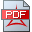A. Einstein, "For us believing in physicists, the distinction between past, present and future is only a stubbornly persistent illusion".

H. Weyl, "We are not surprised that a concrete chunk of nature, taken in its isolated phenomenal existence, challenges our analysis by its in exhaustibility and incompleteness; it is for the sake of completeness, as we have seen, that physics projects what is given onto the background of the possible", (WeH) p. 220.

The two conceptual changes to the inconsistent quantum field theory and the gravity field theory are concerned with

a. the mother of all current types of „elementary particles“, which is an „a priori“ existing „electron“ as pre-requisite of Dirac‘s theory of radiation, (*)

b. the space-time „geometry“ of the GRT modelled as a pair (M,g), where M is a 3+1-dimensional manifold and g is just a metric on M, (**). In other words, (M,g) has no geometric structure all all.

The proposed integrated Hilbert scale based model provide a common quantum element Hilbert space H(-1/2) accompanied by a related quantum energy Hilbert space H(1/2) providing a common (inner product based) metric/norm. The quantum energy Hilbert space can be decomposed into a kinematical compactly and densely embedded sub-Hilbert space H(1) and an orthogonal "ether" energy closed sub-space of H(1/2).

For a conceptual design overview we refer to the section "UFT & MHD".

(*) (FeE): „Dirac‘s theory of radiation is based on a very simple idea; instead of considering an atom and the radiation field with which it interacts as two distinct systems, he treats them as a single system whose energy is the sum of three terms: one representing the energy of the atom, a second representating the electromagnetic energy of the radiation field, and a small term representing the coupling energy of the atom and the radiation field.“

(**) (ChD): The Einstein metric g on M is a nondegenerated, 2-covariant tensor field with the property that at each point one can choose 3+1 vectors e(1),e(2),e(3),e(4) such that g(e(a),e(b))=E(a,b); a,b=0,1,2,3, where E(a,b) is the diagonal matrix with entries -1,1,1,1.Braun K., A geometric Hilbert scale based integrated gravity and quantum field model, Dec 2021 overview

Dec 19, 2021Braun K., A geometric Hilbert scale based Mie electricity field theory accompanied by a complementary 0-point energy space, Sept 2021Braun K., A validation approach of the proposed gravity and quantum field model, June 2021Braun K., A geometric Hilbert scale based gravity and quantum field model, the modelling landscape, May 2021Braun K., A geometric gravity and quantum field model, some royal road markers, April 2021